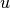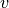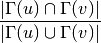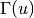Warning

This documents an unmaintained version of NetworkX. Please upgrade to a maintained version and see the current NetworkX documentation.

# jaccard_coefficient¶

Compute the Jaccard coefficient of all node pairs in ebunch.

Jaccard coefficient of nodesandis defined aswheredenotes the set of neighbors of.

Parameters: G (graph) – A NetworkX undirected graph. ebunch (iterable of node pairs, optional (default = None)) – Jaccard coefficient will be computed for each pair of nodes given in the iterable. The pairs must be given as 2-tuples (u, v) where u and v are nodes in the graph. If ebunch is None then all non-existent edges in the graph will be used. Default value: None. piter – An iterator of 3-tuples in the form (u, v, p) where (u, v) is a pair of nodes and p is their Jaccard coefficient. iterator

Examples

>>> import networkx as nx
>>> G = nx.complete_graph(5)
>>> preds = nx.jaccard_coefficient(G, [(0, 1), (2, 3)])
>>> for u, v, p in preds:
...     '(%d, %d) -> %.8f' % (u, v, p)
...
'(0, 1) -> 0.60000000'
'(2, 3) -> 0.60000000'


References

  D. Liben-Nowell, J. Kleinberg. The Link Prediction Problem for Social Networks (2004). http://www.cs.cornell.edu/home/kleinber/link-pred.pdf